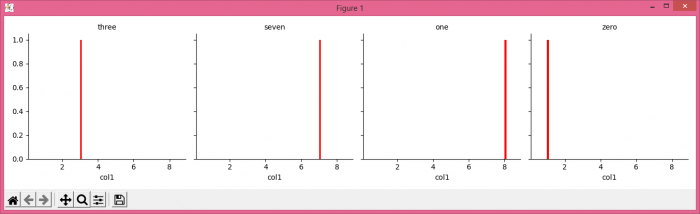# Customizing annotation with Seaborn's FacetGrid

MatplotlibPythonData Visualization

To customizing annotation with seaborn's face grid, we can take following steps −

• Set the figure size and adjust the padding between and around the subplots.
• Create a data frame with col1 and col2 columns.
• Multi-plot grid for plotting conditional relationships.
• Apply a plotting function to each facet's subset of the data.
• Set the title of each grids.
• To display the figure, use show() method.

## Example

import pandas as pd
import seaborn as sns
from matplotlib import pyplot as plt

plt.rcParams["figure.figsize"] = [7.50, 3.50]
plt.rcParams["figure.autolayout"] = True

df = pd.DataFrame({'col1': [3, 7, 8, 1], 'col2': ["three", "seven", "one", "zero"]})
g = sns.FacetGrid(data=df, col='col2', height=3.5)
g.map(plt.hist, 'col1', color='red', lw=0)
g.set_titles('{col_name}')

plt.show()

## Output